# Ruler

How far from Peter stands 2m hight John? Petr is looking to John over ruler that keeps at arm's distant 60 cm from the eye and on the ruler John measured the height of 15 mm.

Correct result:

x =  80 m

#### Solution:

x / 2 = 0.60 / 0.015

x = 80

Our simple equation calculator calculates it.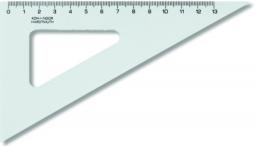We would be pleased if you find an error in the word problem, spelling mistakes, or inaccuracies and send it to us. Thank you!Tips to related online calculators
Do you have a linear equation or system of equations and looking for its solution? Or do you have quadratic equation?
Do you want to convert length units?

#### You need to know the following knowledge to solve this word math problem:

We encourage you to watch this tutorial video on this math problem:

## Next similar math problems:

• Triangle P2Can triangle have two right angles?
• Bisectors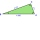As shown, in △ ABC, ∠C = 90°, AD bisects ∠BAC, DE⊥AB to E, BE = 2, BC = 6. Find the perimeter of triangle △ BDE.
• Complementary angles 2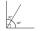Two complementary angles are (x+4) and (2x - 7) find the value of x
• Largest angle of the triangleWhat is the largest angle of the triangle if the second angle is 10° greater than twice the first and the third is 30° smaller than the second?
• AnglesIn the triangle ABC, the ratio of angles is: a:b = 4: 5. The angle c is 36°. How big are the angles a, b?
• CablewayCableway has a length of 1800 m. The horizontal distance between the upper and lower cable car station is 1600 m. Calculate how much meters altitude is higher upper station than the base station.
• Railway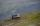Between points A, B, whose horizontal distance is 1.5 km railway line has 8promile climb. Between points B, C with horizontal distance of 900 m is climb 14promile. Calculate differences of altitudes between points A and C.
• Chimney and treeCalculate the height of the factory chimney, which casts a shadow 6.5 m long in the afternoon. At the same time, a 6 m high tree standing near it casts a shadow 25 dm long.
• A boyA boy of height 1.7m is standing 30m away from flag staff on the same level ground . He observes that the angle of deviation of the top of flag staff is 30 degree. Calculate the height of flag staff.Mast has 13 m long shadow on a slope rising from the mast foot in the direction of the shadow angle at angle 15°. Determine the height of the mast, if the sun above the horizon is at angle 33°. Use the law of sines.
• SimilarityAre two right triangles similar to each other if the first one has a acute angle 70° and second one has acute angle 20°?
• The triangles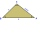The triangles KLM and ABC are given, which are similar to each other. Calculate the lengths of the remaining sides of the triangle KLM, if the lengths of the sides are a = 7 b = 5.6 c = 4.9 k = 5
• A drone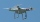A flying drone aimed the area for an architect. He took off perpendicularly from point C to point D. He was at a height of 300 m above the plane of ABC. The drone from point D pointed at a BDC angle of 43°. Calculate the distance between points C and B in
• ThalesThales is 1 m from the hole. The eyes are 150 cm above the ground and look into the hole with a diameter of 120 cm as shown. Calculate the depth of the hole.Miro stands under a tree and watching its shadow and shadow of the tree. Miro is 180 cm tall and its shade is 1.5 m long. The shadow of the tree is three times as long as Miro's shadow. How tall is the tree in meters?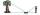How far must Paul place a mirror to see the top of the tower 12 m high? The height of Paul's eyes above the horizontal plane is 160 cm and Paul is from the tower distant 20 m.Tree perpendicular to the horizontal surface has a shadow 8.32 meters long. At the same time meter rod perpendicular to the horizontal surface has shadow 64 cm long. How tall is tree?